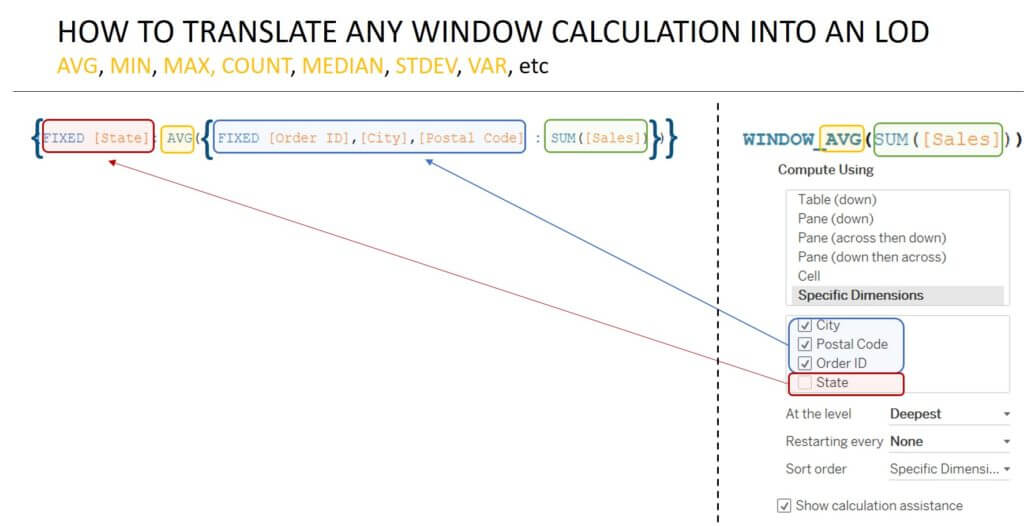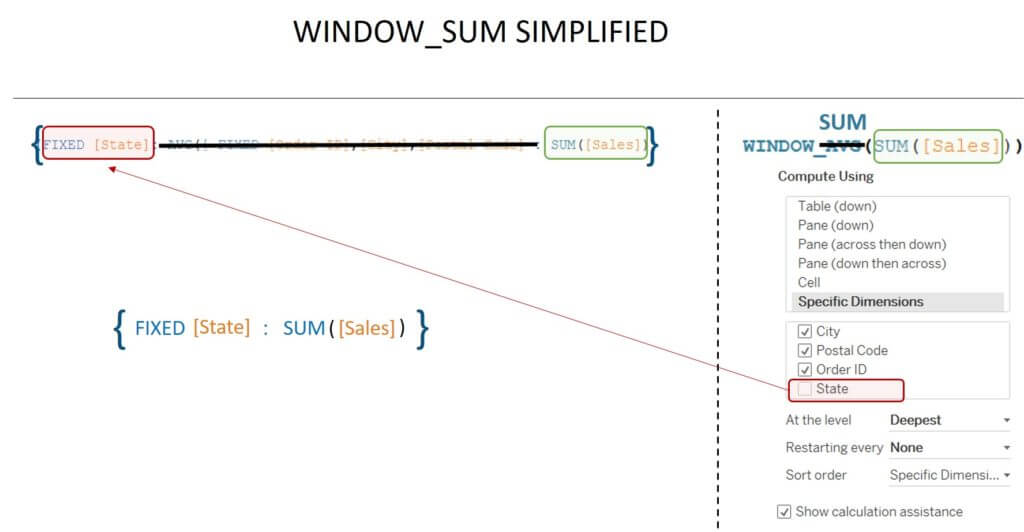## Table Calculations vs Level of Detail Calculations Series 1: Converting Window Calcs to LODs

by Mina Ozgen

Welcome to my simple post on converting window calculations into level of detail calculations (LOD). I will explain a universal logic for converting window calculations into LODs.

The idea behind this is that any table calculation can be converted into an LOD by using a nested LOD.It is simplest to just use the diagram above to understand but I will add a bit of text below for assistance.

The inner LOD essentially recreates the necessary view (ticked dimensions) to get the correct AGG([Measure]) that we normally pass into the window calculation (in this case (SUM([Sales])) .

The outer LOD computes the equivalent of the WINDOW_AGG(AGG([Measure])), for each unique dimension/set of dimensions (unticked dimensions).

#### Simplifications

Whilst the case above builds a logic to convert any Window Calculation into an LOD, these are not necessarily the shortest equivalent LOD. For example in converting WINDOW_SUM(SUM([Measure])) it is not necessary to nest as there would be a summation of a nested summations (and these are equal to just performing one summation since it does not matter what order everything is summed altogether).

In this case, our LOD becomes {FIXED Dimensions : Sum(Measure)}.The nested operations do become important when we talk about any other operations, however (as the order of operations is important).

#### Glossary

View – The summarised table/chart displayed on a tableau worksheet by adding dimensions and measure to shelves and cards.
LOD – Level of Detail Calculation
AGG – Aggregation
Window Calculation – An aggregation performed on the table feeding the view.Mina Ozgen

Mon 02 Oct 2017

Thu 24 Aug 2017

Wed 16 Aug 2017

Wed 16 Aug 2017